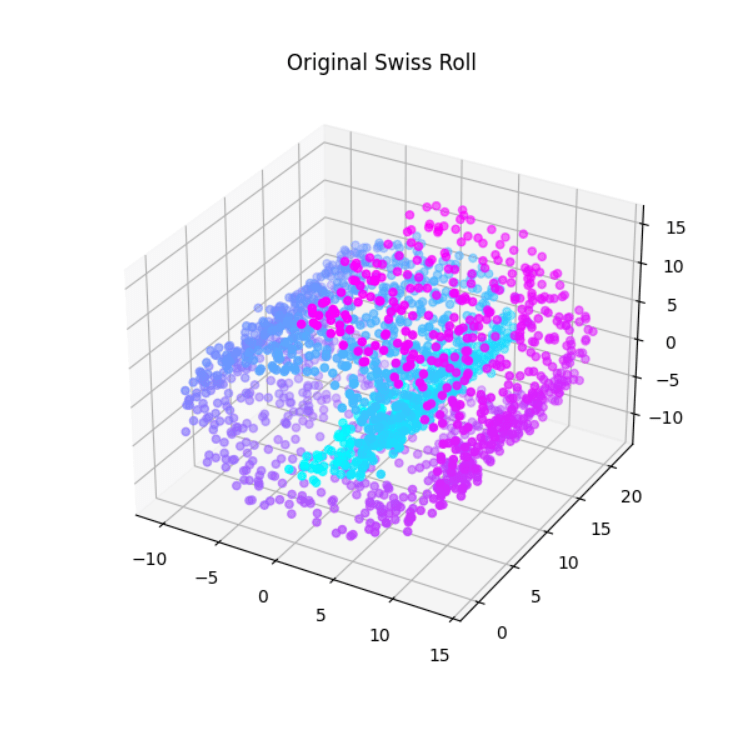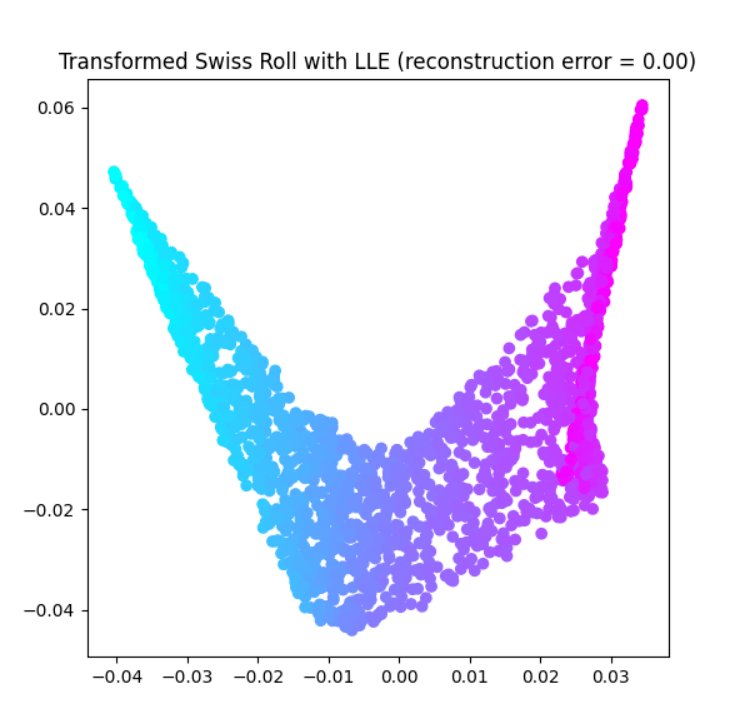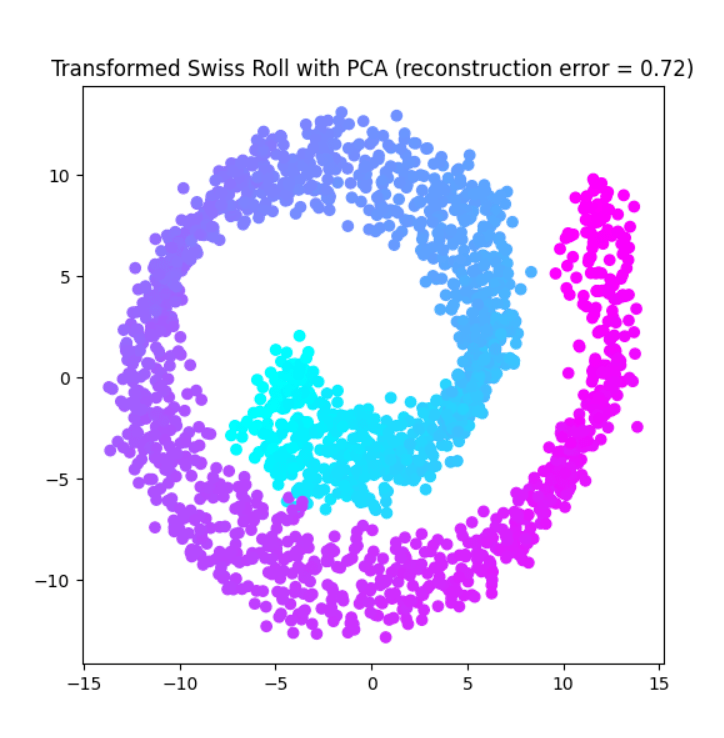# Swiss Roll Reduction with LLE in Scikit Learn

This article discusses the concept of dimensionality reduction, specifically using the Swiss Roll dataset and the Locally Linear Embedding (LLE) algorithm. The article discusses the process involved in performing Swiss Roll reduction with LLE, including the steps of loading and preprocessing the data, fitting the LLE model, and visualizing the reduced data. The article also provides a Python implementation of the process, with a code walkthrough and output examples. The article concludes with observations on the effectiveness of LLE in reducing the dimensionality of the Swiss Roll dataset.

### What is Swiss Roll?

The Swiss roll is a toy dataset in scikit learn that is commonly used for testing and demonstrating nonlinear dimensionality reduction algorithms. It consists of a set of points in three dimensions, arranged in a “roll” shape, such that the points on the roll are mapped to a two-dimensional plane in a nonlinear fashion. The points on the Swiss roll are often colored so that the resulting plot of the reduced data can be visualized more easily.

The Swiss roll dataset is often used because it is easy to generate and visualize, and because it exhibits a nonlinear structure that is not captured by linear dimensionality reduction methods such as PCA. It is also a useful benchmark for comparing the performance of different nonlinear dimensionality reduction algorithms.

### What is Swiss Roll reduction?

Swiss roll reduction is the process of reducing the dimensionality of the Swiss roll dataset from three dimensions to two or fewer dimensions, using a dimensionality reduction algorithm. The goal of this process is to represent the original data in a lower-dimensional space while preserving as much of the underlying structure of the data as possible.

There are many techniques that can be used for Swiss roll reduction, including linear techniques such as Principal Component Analysis (PCA) and nonlinear techniques such as Locally Linear Embedding (LLE), Isomap, and t-SNE. Each of these techniques has its own strengths and weaknesses and can be more or less effective depending on the characteristics of the data and the goals of the analysis.

In general, linear techniques such as PCA are faster and more computationally efficient, but may not be able to capture complex nonlinear structures in the data. Nonlinear techniques, on the other hand, can capture more complex structures but may be slower and more computationally intensive. Choosing the right technique for Swiss roll reduction will depend on the specific goals of the analysis and the characteristics of the data.

### What is Locally Linear Embedding (LLE)?

Locally Linear Embedding (LLE) is a method for nonlinear dimensionality reduction that is based on the idea of modeling the local structure of the data by reconstructing it from a linear combination of its neighbors. The idea behind LLE is to find a low-dimensional representation of the data that preserves the relationships between nearby points while ignoring the relationships between more distant points.

To perform LLE, the algorithm first selects a set of neighbors for each point in the data, based on some measure of similarity such as Euclidean distance. It then fits a linear model to these neighbors, using the original high-dimensional coordinates as the input variables and the low-dimensional coordinates as the output variables. This allows the algorithm to reconstruct each point in the data as a linear combination of its neighbors, using the low-dimensional coordinates as the coefficients. The low-dimensional coordinates are then optimized to minimize the reconstruction error.

LLE has a number of attractive properties, including its ability to handle high-dimensional data and its ability to preserve the local structure of the data. However, it can be sensitive to the choice of the number of neighbors and the dimensionality of the output space, and may not perform well on data with a complex global structure.

#### The process involved in Swiss Roll reduction with LLE?

Here is a general outline of the process involved in reducing the dimensionality of the Swiss roll dataset using Locally Linear Embedding (LLE):

1. Load the Swiss roll dataset: The first step is to load the Swiss roll dataset and store it in a variable X. This can be done using scikit-learn’s make_swiss_roll function.
2. Fit the LLE model to the data: Next, you can use scikit-learn’s LocallyLinearEmbedding class to fit an LLE model to the data. This requires specifying the number of dimensions in the reduced data (n_components) and the number of neighbors to use in the reconstruction process (n_neighbors). You can also specify other optional parameters, such as the distance metric to use for selecting neighbors.
3. Transform the data: Once the LLE model has been fit to the data, you can use the transform method to reduce the dimensionality of the data. This will return a new set of coordinates for the data points, with the specified number of dimensions.
4. Optionally, compute the reconstruction error of the model using the reconstruction_error_ attribute of the LocallyLinearEmbedding object.
5. Visualize the reduced data: Finally, you can visualize the reduced data using a scatter plot or other suitable visualization technique. This can help you understand the structure of the data and assess the effectiveness of the dimensionality reduction process. Plot the original Swiss Roll data in 3D and the transformed data in the lower-dimensional space using Matplotlib.

Here is an example of a comprehensive Python code that demonstrates how to use Locally Linear Embedding (LLE) for Swiss roll reduction in scikit-learn, including pre-processing and comparison with PCA, and visualization steps

## Python3

 `import` `matplotlib.pyplot as plt ` `from` `mpl_toolkits.mplot3d ``import` `Axes3D ` `from` `sklearn.datasets ``import` `make_swiss_roll ` `from` `sklearn.manifold ``import` `LocallyLinearEmbedding ` `from` `sklearn.decomposition ``import` `PCA ` ` `  `# Generate the data ` `X, y ``=` `make_swiss_roll(n_samples``=``2000``, noise``=``0.5``) ` ` `  `# Fit the model with LLE ` `lle ``=` `LocallyLinearEmbedding(n_components``=``2``, n_neighbors``=``10``) ` `X_lle ``=` `lle.fit_transform(X) ` `reconstruction_error_lle ``=` `lle.reconstruction_error_ ` ` `  `# Fit the model with PCA ` `pca ``=` `PCA(n_components``=``2``) ` `X_pca ``=` `pca.fit_transform(X) ` `reconstruction_error_pca ``=` `sum``(pca.explained_variance_ratio_) `

In this code, we are using the make_swiss_roll function from scikit-learn to generate a synthetic 3D dataset, known as the Swiss Roll. The Swiss Roll is a toy dataset used for demonstrating nonlinear dimensionality reduction techniques. It consists of a rolled-up 2D plane, with points on the plane randomly distributed and colored.

## Python3

 `import` `matplotlib.pyplot as plt ` `from` `mpl_toolkits.mplot3d ``import` `Axes3D ` `from` `sklearn.datasets ``import` `make_swiss_roll ` `from` `sklearn.manifold ``import` `LocallyLinearEmbedding ` `from` `sklearn.decomposition ``import` `PCA ` ` `  `# Generate the data ` `X, y ``=` `make_swiss_roll(n_samples``=``2000``, ` `                       ``noise``=``0.5``) `

Next, we apply two different dimensionality reduction techniques to the Swiss Roll dataset: Locally Linear Embedding (LLE) and Principal Component Analysis (PCA), PCA is applied for comparison purposes with LLE. LLE is a nonlinear dimensionality reduction method that seeks to preserve the local structure of the data by reconstructing each point as a linear combination of its neighbors. PCA, on the other hand, is a linear dimensionality reduction method that seeks to find the directions of maximum variance in the data and projects the data onto a new lower-dimensional space along these directions.

To apply LLE, we use the LocallyLinearEmbedding class from scikit-learn. We initialize the class with the number of dimensions we want to reduce the data to (in this case, 2), and the number of neighbors to use for the reconstruction of each point. Then, we fit the model to the data using the fit_transform method, which returns the transformed data in the lower-dimensional space. We also compute the reconstruction error of the LLE model using the reconstruction_error_ attribute of the LocallyLinearEmbedding object.

## Python3

 `# Fit the model with LLE ` `lle ``=` `LocallyLinearEmbedding(n_components``=``2``, ` `                             ``n_neighbors``=``10``) ` `X_lle ``=` `lle.fit_transform(X) ` `reconstruction_error_lle ``=` `lle.reconstruction_error_ `

To apply PCA, we use the PCA class from scikit-learn. We initialize the class with the number of dimensions we want to reduce the data to (in this case, 2) and fit the model to the data using the fit_transform method, which returns the transformed data in the lower-dimensional space. We also compute the reconstruction error of the PCA model by summing up the explained variance ratios of the selected components.

## Python3

 `# Fit the model with PCA ` `pca ``=` `PCA(n_components``=``2``) ` `X_pca ``=` `pca.fit_transform(X) ` `reconstruction_error_pca ``=` `sum``(pca.explained_variance_ratio_) `

Finally, we plot the original Swiss Roll data in 3D, the transformed Swiss Roll data using LLE in 2D, and the transformed Swiss Roll data using PCA in 2D. The plots show the data points colored by their original position on the rolled-up plane, so we can see how well the dimensionality reduction techniques have preserved the structure of the data. The plot titles also display the reconstruction error for each method.

## Python3

 `# Plot the original data ` `fig ``=` `plt.figure(figsize``=``(``6``, ``6``)) ` `ax ``=` `fig.add_subplot(``111``, projection``=``'3d'``) ` `ax.scatter(X[:, ``0``], X[:, ``1``], X[:, ``2``], ` `           ``c``=``y, cmap``=``plt.cm.cool) ` `plt.title(``'Original Swiss Roll'``) ` `plt.show() `

Output:In the plot of the original Swiss Roll data, you should see a 3D scatterplot with points colored by their original position on the rolled-up plane. The points should form a spiral shape, with the colors changing smoothly along the spiral. This represents the original structure of the data, with points on the plane randomly distributed and colored.

## Python3

 `# Plot the transformed data with LLE ` `fig ``=` `plt.figure(figsize``=``(``6``, ``6``)) ` `plt.scatter(X_lle[:, ``0``], X_lle[:, ``1``], c``=``y, cmap``=``plt.cm.cool) ` `plt.title( ` `    ``f'Transformed Swiss Roll with LLE\ ` `    ``(reconstruction error ``=` `{reconstruction_error_lle:.``2f``})') ` `plt.show() `

Output:In the plot of the transformed Swiss Roll data using LLE, you should see a 2D scatterplot with points colored by their original position on the rolled-up plane.

## Python3

 `# Plot the transformed data with PCA ` `fig ``=` `plt.figure(figsize``=``(``6``, ``6``)) ` `plt.scatter(X_pca[:, ``0``], X_pca[:, ``1``], ` `            ``c``=``y, cmap``=``plt.cm.cool) ` `plt.title( ` `    ``f'Transformed Swiss Roll with PCA \ ` `    ``(reconstruction error ``=` `{reconstruction_error_pca:.``2f``})') ` ` `  `plt.show() `

Output:In the plot of the transformed Swiss Roll data using PCA, you should see a 2D scatterplot with points colored by their original position on the rolled-up plane. The points should not necessarily form a spiral shape, as PCA is a linear dimensionality reduction method and may not be able to capture the nonlinear structure of the data. However, the points should still be somewhat ordered by color, indicating that PCA has been able to preserve some of the structure of the data by finding the directions of maximum variance.

Overall, the output plots allow us to compare the performance of LLE and PCA in preserving the structure of the Swiss Roll data after dimensionality reduction. The reconstruction error for each method, displayed in the plot titles, provides a quantitative measure of this performance. A lower reconstruction error indicates better preservation of the original structure of the data.

Whether you're preparing for your first job interview or aiming to upskill in this ever-evolving tech landscape, GeeksforGeeks Courses are your key to success. We provide top-quality content at affordable prices, all geared towards accelerating your growth in a time-bound manner. Join the millions we've already empowered, and we're here to do the same for you. Don't miss out - check it out now!

Previous
Next